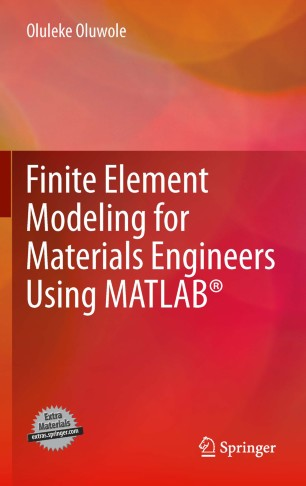# Finite Element Modeling for Materials Engineers Using MATLAB®

• Oluleke OluwoleBook

1. Front Matter
Pages i-xi
2. Oluleke Oluwole
Pages 1-1
3. Oluleke Oluwole
Pages 3-12
4. Oluleke Oluwole
Pages 13-33
5. Oluleke Oluwole
Pages 35-58
6. Oluleke Oluwole
Pages 59-72
7. Oluleke Oluwole
Pages 73-83
8. Oluleke Oluwole
Pages 85-104
9. Back Matter
Pages 105-120

### Introduction

The finite element method is often used for numerical computation in the applied sciences. It makes a major contribution to the range of numerical methods used in the simulation of systems and irregular domains, and its importance today has made it an important subject of study for all engineering students.

While treatments of the method itself can be found in many traditional finite element books, Finite Element Modeling for Materials Engineers Using MATLAB® combines the finite element method with MATLAB to offer materials engineers a fast and code-free way of modeling for many materials processes.

Finite Element Modeling for Materials Engineers Using MATLAB® covers such topics as:

• developing a weak formulation as a prelude to obtaining the finite element equation,
• interpolation functions,
• derivation of elemental equations, and
• use of the Partial Differential Equation Toolbox™.

Exercises are given based on each example and m-files based on the examples are freely available to readers online.

Researchers, advanced undergraduate and postgraduate students, and practitioners in the fields of materials and metallurgy will find Finite Element Modeling for Materials Engineers Using MATLAB® a useful guide to using MATLAB for engineering analysis and decision-making.

### Keywords

Finite Element Finite Element Modeling MATLAB Materials Engineering Materials Processes

#### Authors and affiliations

• Oluleke Oluwole
• 1